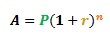# Finding the Time Period for Compound Interest

by Nirmal
(Dubai)

##### Question

in what time the compound interest on Rs800 at 20% per annum will amount to Rs1152 if compounded annually?

STEP 1
The compound interest formula is given below:Where:

• A is the total amount of money (including interest) after n years

• P is the principal (the amount money borrowed or invested)

• r is the interest rate (per year or per annum)

• n is the loan or investment duration in years

STEP 2
Thus, let us substitute the values we have into the formula:
1152 = 800(1+0.2)^n

STEP 3
We can then proceed to solve the equation:
1152/800 = (1.2)^n
1.44 = (1.2)^n
1.44 = (1.2)^2

Thus, it takes two years for the compound interest to amount to Rs1152.

You can also refer to our tutorial on how to calculate compound interest.

### Comments for Finding the Time Period for Compound Interest

Average RatingOct 13, 2020 Ratingfinal answer NEW by: Anonymous How did you get the two years?